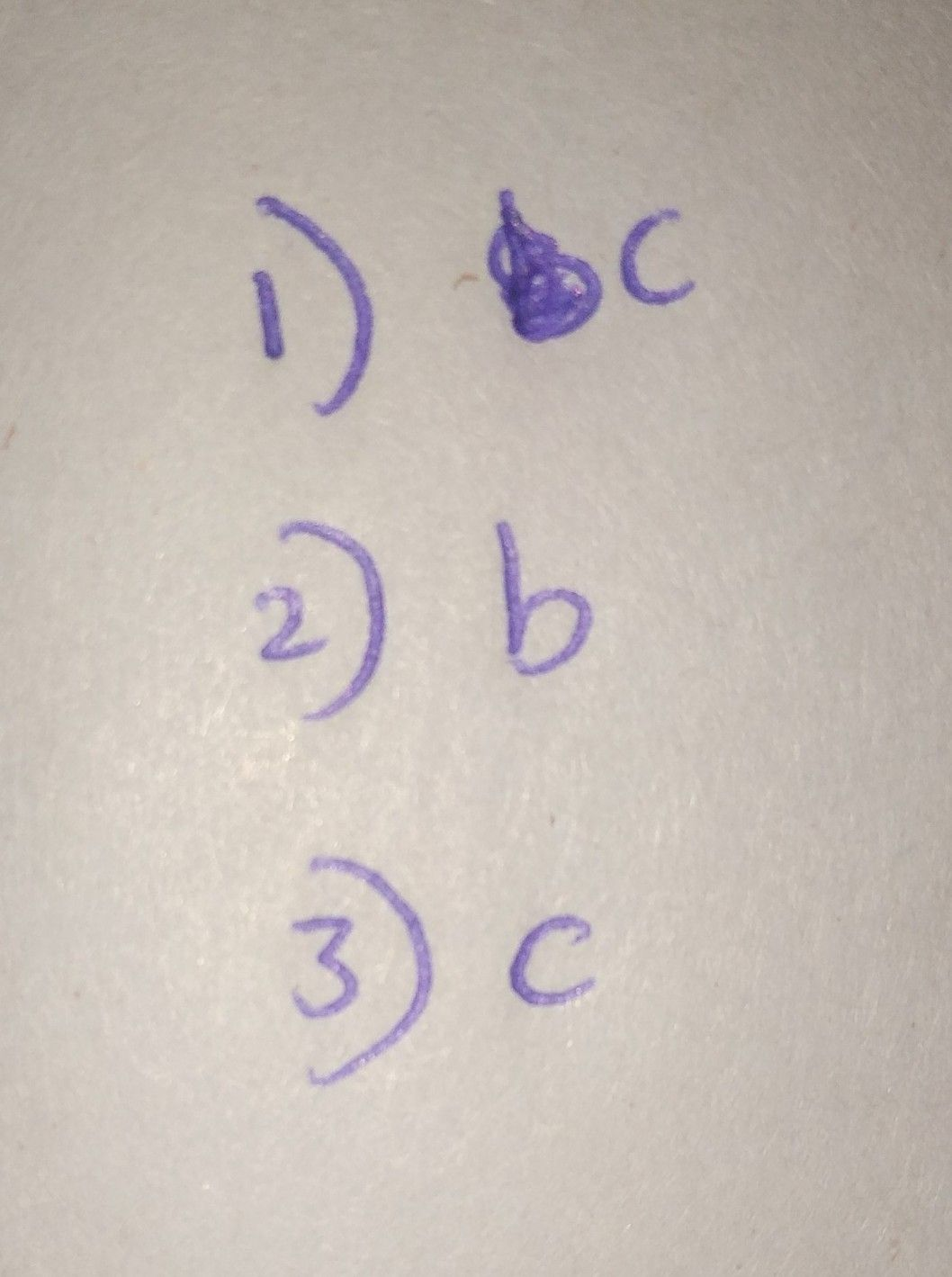Symbol
ProblemChoose the letter of the best answer. Write the chosen letter on a separate sheet of paper. $11$ This expresses a relationship between the input and the output and can be represented through a table of values, graph, and equation, a. logarithmic equation c. logarithmic inequality b. logarithmic function d. none of these $2$ Which of the following $13$ an example of $a0$ $logarthmic$ function? $a$ $log _{,}27=log _{,}x$ $c$ $log _{2}x<10$ $1$ $b$ $y=log _{2}\left(2x-1\right)$ $d$ $log _{2}x=og$ $2$ $3.$ It is $an$ inequality involving logarithms. $a$ logarithmic equation $c1$ $ogan1nmtc$ inequality b. logarithmic function $1$ $1$ $-$ logarithmic expression
10th-13th grade
Other
Search count: 138
SolutionQanda teacher - AravindStudent
with solution please
you know?